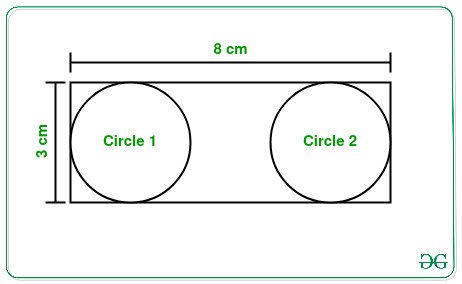# Number of largest circles that can be inscribed in a rectangle

• Last Updated : 21 Apr, 2021

Given two integers L and B representing the length and breadth of a rectangle, the task is to find the maximum number of largest possible circles that can be inscribed in the given rectangle without overlapping.

Examples:

Input: L = 3, B = 8
Output: 2
Explanation:From the above figure it can be clearly seen that the largest circle with a diameter of 3 cm can be inscribed in the given rectangle.
Therefore, the count of such circles is 2.

Input: L = 2, B = 9
Output: 4

Approach: The given problem can be solved based on the following observations:

• The largest circle that can be inscribed in a rectangle will have diameter equal to the smaller side of the rectangle.
• Therefore, the maximum number of such largest circles possible is equal to ( Length of the largest side ) / ( Length of the smallest side ).

Therefore, from the above observation, simply print the value of ( Length of the largest side ) / ( Length of the smallest side ) as the required result.

Below is the implementation of the above approach:

## C++

 `// C++ program for the above approach` `#include ``using` `namespace` `std;` `// Function to count the number of``// largest circles in a rectangle``int` `totalCircles(``int` `L, ``int` `B)``{``    ``// If length exceeds breadth``    ``if` `(L > B) {` `        ``// Swap to reduce length``        ``// to smaller than breadth``        ``int` `temp = L;``        ``L = B;``        ``B = temp;``    ``}` `    ``// Return total count``    ``// of circles inscribed``    ``return` `B / L;``}` `// Driver Code``int` `main()``{``    ``int` `L = 3;``    ``int` `B = 8;``    ``cout << totalCircles(L, B);` `    ``return` `0;``}`

## Java

 `// Java program for the above approach``import` `java.io.*;``import` `java.util.*;``class` `GFG``{` `  ``// Function to count the number of``  ``// largest circles in a rectangle``  ``static` `int` `totalCircles(``int` `L, ``int` `B)``  ``{``    ``// If length exceeds breadth``    ``if` `(L > B) {` `      ``// Swap to reduce length``      ``// to smaller than breadth``      ``int` `temp = L;``      ``L = B;``      ``B = temp;``    ``}` `    ``// Return total count``    ``// of circles inscribed``    ``return` `B / L;``  ``}` `  ``// Driver Code``  ``public` `static` `void` `main(String[] args)``  ``{``    ``int` `L = ``3``;``    ``int` `B = ``8``;``    ``System.out.print(totalCircles(L, B));``  ``}``}` `// This code is contributed by susmitakundugoaldanga.`

## Python3

 `# Python3 program for the above approach` `# Function to count the number of``# largest circles in a rectangle``def` `totalCircles(L, B) :``    ` `    ``# If length exceeds breadth``    ``if` `(L > B) :` `        ``# Swap to reduce length``        ``# to smaller than breadth``        ``temp ``=` `L``        ``L ``=` `B``        ``B ``=` `temp``    ` `    ``# Return total count``    ``# of circles inscribed``    ``return` `B ``/``/` `L` `# Driver Code``L ``=` `3``B ``=` `8``print``(totalCircles(L, B))` `# This code is contributed by splevel62.`

## C#

 `// C# program to implement``// the above approach``using` `System;``public` `class` `GFG``{` `  ``// Function to count the number of``  ``// largest circles in a rectangle``  ``static` `int` `totalCircles(``int` `L, ``int` `B)``  ``{``    ``// If length exceeds breadth``    ``if` `(L > B) {` `      ``// Swap to reduce length``      ``// to smaller than breadth``      ``int` `temp = L;``      ``L = B;``      ``B = temp;``    ``}` `    ``// Return total count``    ``// of circles inscribed``    ``return` `B / L;``  ``}`  `  ``// Driver Code``  ``public` `static` `void` `Main(String[] args)``  ``{``    ``int` `L = 3;``    ``int` `B = 8;``    ``Console.Write(totalCircles(L, B));``  ``}``}` `// This code is contributed by souravghosh0416.`

## Javascript

 ``

Output:

`2`

Time Complexity: O(1)
Auxiliary Space: O(1)

My Personal Notes arrow_drop_up